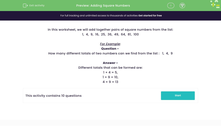### Comprehensive & curriculum aligned

In this worksheet, students answer questions related to the addition of two square numbers.Key stage:  KS 2

Curriculum topic:   Number: Multiplication and Division

Curriculum subtopic:   Recognise Square/Cube Numbers

Popular topics:   Ratio worksheets, Numbers worksheets, Square Numbers worksheets

Difficulty level:#### Worksheet Overview

In this worksheet, we will add together pairs of square numbers from the list:
1,  4,  9,  16,  25,  36,  49,  64,  81,  100

For Example:

Question -
How many different totals of two numbers can we find from the list :   1,  4,  9

Different totals that can be formed are:

1 + 4 = 5,

1 + 9 = 10,

4 + 9 = 13

### What is EdPlace?

We're your National Curriculum aligned online education content provider helping each child succeed in English, maths and science from year 1 to GCSE. With an EdPlace account you’ll be able to track and measure progress, helping each child achieve their best. We build confidence and attainment by personalising each child’s learning at a level that suits them.

Get started